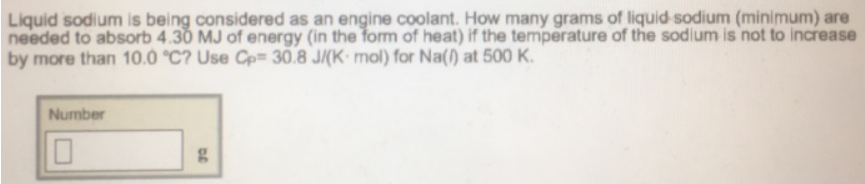# Problem: Liquid sodium is being considered as an engine coolant. How many grams of liquid sodium (minimum) are needed to absorb 4.30 MJ of energy (in the form of heat) if the temperature of the sodium is not to increase by more than 10.0°C? Use Cp = 30.8 J/(K • mol) for Na(l) at 500 K.

###### FREE Expert Solution
96% (424 ratings)###### Problem Details

Liquid sodium is being considered as an engine coolant. How many grams of liquid sodium (minimum) are needed to absorb 4.30 MJ of energy (in the form of heat) if the temperature of the sodium is not to increase by more than 10.0°C? Use Cp = 30.8 J/(K • mol) for Na(l) at 500 K.